# Case Based Questions Test: Electricity - 3

## 15 Questions MCQ Test Online MCQ Tests for Class 10 | Case Based Questions Test: Electricity - 3

Description
Attempt Case Based Questions Test: Electricity - 3 | 15 questions in 30 minutes | Mock test for Class 10 preparation | Free important questions MCQ to study Online MCQ Tests for Class 10 for Class 10 Exam | Download free PDF with solutions
QUESTION: 1

### In the given circuit, connect a nichrome wire of length ‘L’ between points X and Y and note the ammeter reading Answer the questions: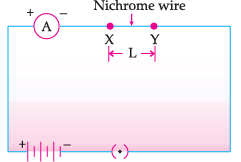Q. When this experiment is repeated by inserting another nichrome wire of the same thickness but twice the length (2L), what changes are observed in the ammeter reading ?

Solution: ammeter reading will decrease (becomes half) Explanation: The ammeter reading will decrease (becomes half). This is because with the increase in length, resistance of the circuit increases, hence current decreases.
QUESTION: 2

### n the given circuit, connect a nichrome wire of length ‘L’ between points X and Y and note the ammeter reading Answer the questions:Q. The instrument used to measure current is ____________.

Solution: An instrument called ammeter measures electric current in a circuit. It is always connected in series in a circuit through which the current is to be measured. Also ammeter has negligible resistance, thus no voltage gets dropped across it.
QUESTION: 3

### n the given circuit, connect a nichrome wire of length ‘L’ between points X and Y and note the ammeter reading Answer the questions:Q. State the changes that are observed in the ammeter reading if we double the area of the cross section without changing the length in the above experiment.

Solution:

The ammeter reading will increase (becomes two times). This is because as area increases, resistance decreases and hence current increases.

QUESTION: 4

n the given circuit, connect a nichrome wire of length ‘L’ between points X and Y and note the ammeter reading Answer the questions:Q. When nichrome and copper wire of same length and same radius are connected in series and current I is passed through them. Which wire gets heated up more?

Solution: Resistance of nichrome is greater than copper wire of the same length and same radius, because the former has greater resistivity.

In series, Power loss P = I2R. Because of higher resistance power loss and heating up of nichrome wire is more than that of copper.

QUESTION: 5

n the given circuit, connect a nichrome wire of length ‘L’ between points X and Y and note the ammeter reading Answer the questions:Q. In a circuit two resistors of 5 Ω and 10 Ω are connected in series. Compare the current passing through the two resistors.

Solution: In a series connection of resistors, the same current passes through all the resistors. Hence, the current will be the same. Ratio of the currents will be 1 : 1.
QUESTION: 6

Observe the following table and answer the questions. Electrical resistivities of some substances, at 20°C are given as follows: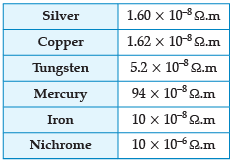Q. Which is a better conductor of electric current:

Solution: Metals are good conductors of electricity. silver is found in in pure form from the earth. so In the given options all are metals. Among them silver is the best conductor of electricity after second best conductor of electricity is gold.
QUESTION: 7

Observe the following table and answer the questions. Electrical resistivities of some substances, at 20°C are given as follows:Q. Series arrangement is not used for domestic circuits because:

Solution: In series circuits voltage is divided. Each component of a series circuit receives a small voltage so the amount of current decreases and the device becomes hot and does not work properly. Hence, the series arrangement is not used in domestic circuits.
QUESTION: 8

Observe the following table and answer the questions. Electrical resistivities of some substances, at 20°C are given as follows:Q. Which element will be used for electrical transmission lines:

Solution: Copper wires can be used for electrical transmission lines because copper has lower resistivity. Power loss in the form of heat is minimum when electricity is passed through copper wires.
QUESTION: 9

Observe the following table and answer the questions. Electrical resistivities of some substances, at 20°C are given as follows:Q. If the resistance is to be increased, then the resistors are to be increased in:

Solution: Equivalent resistance is maximum and current flow is minimum when number of resistances are connected in series. Req = R1 + R2 + R3 ; where R1 ,R2 ,R3 are resistances.
QUESTION: 10

Observe the following table and answer the questions. Electrical resistivities of some substances, at 20°C are given as follows:Q. Nichrome is used in the heating elements of electric heating device because:

Solution: Nichrome is used for making heating elements of electrical appliances. Because nichrome does not oxidize and burn easily at high temperature i.e.it has higher melting and boiling point than metals. Thus it does not melt even when a large amount of heat is produced due to passage of current. Moreover, It has higher resistivity and consequently a higher resistance.Therefore, it will resist the flow of charges more, and lead to development of heat faster.
QUESTION: 11

Study the given table and answer the questions :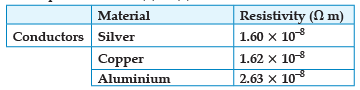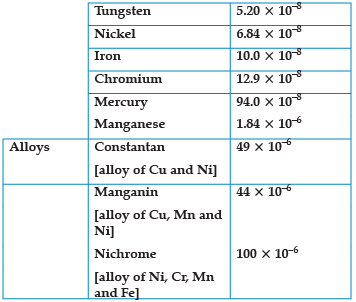Q. Which is a better conductor:

Solution:

Nickel is a fairly good conductor of electricity and heat and is one of only four elements (cobalt, iron, nickel and gadolinium) that are ferromagnetic (magnetized easily) at room temperature.

QUESTION: 12

Study the given table and answer the questions :Q. What happens to resistance of a conductor when its area of cross section is increased?

Solution: On increasing the area of cross-section, resistance decreases. This is because resistance is inversely proportional to area.
QUESTION: 13

Study the given table and answer the questions :Q. Element used to make heating element of electric geyser:

Solution: Nichrome: Most resistance wire heating elements usually use nichrome 80/20 (80% Nickel, 20% Chromium) wire, ribbon, or strip. Nichrome 80/20 is an ideal material, because it has relatively high resistance and forms an adherent layer of chromium oxide when it is heated for the first time.
QUESTION: 14

Study the given table and answer the questions :Q. A given length of a wire is doubled and this process is repeated once again. The resistance of wire becomes:

Solution: Length becomes one-fourth of the original length and area of cross-section becomes four times that of original.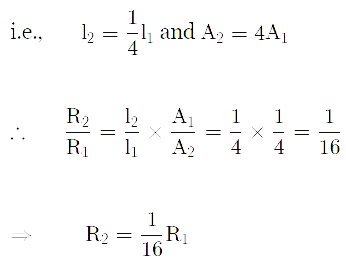So, new resistance is (1/16)th of original resistance.

QUESTION: 15

Study the given table and answer the questions :Q. Element used to make filament of incandescent bulb:

Solution: Incandescent light bulbs have filaments which are made mainly from the element tungsten, the metal element used for the filaments in incandescent light bulbs.Use Code STAYHOME200 and get INR 200 additional OFF Use Coupon Code